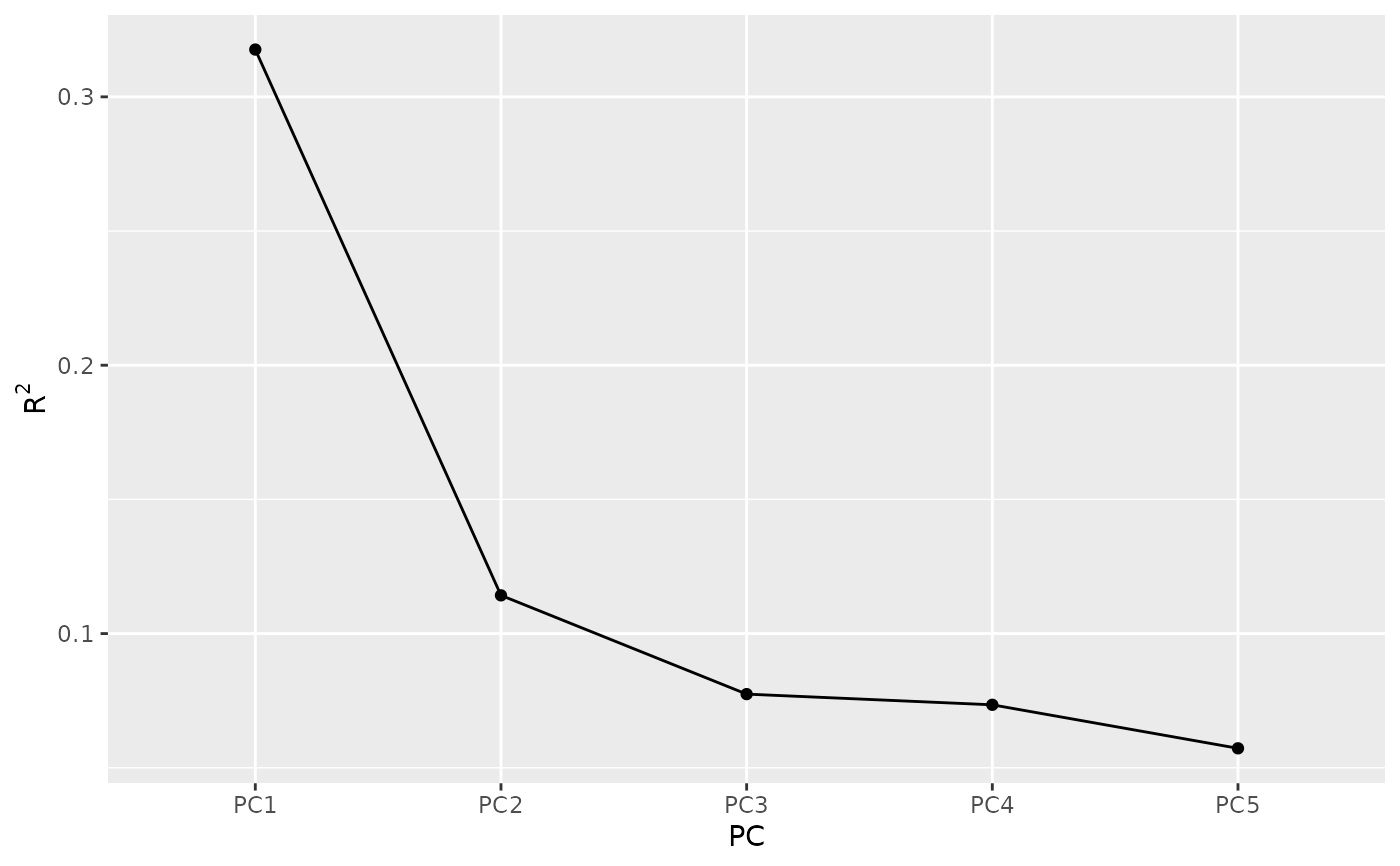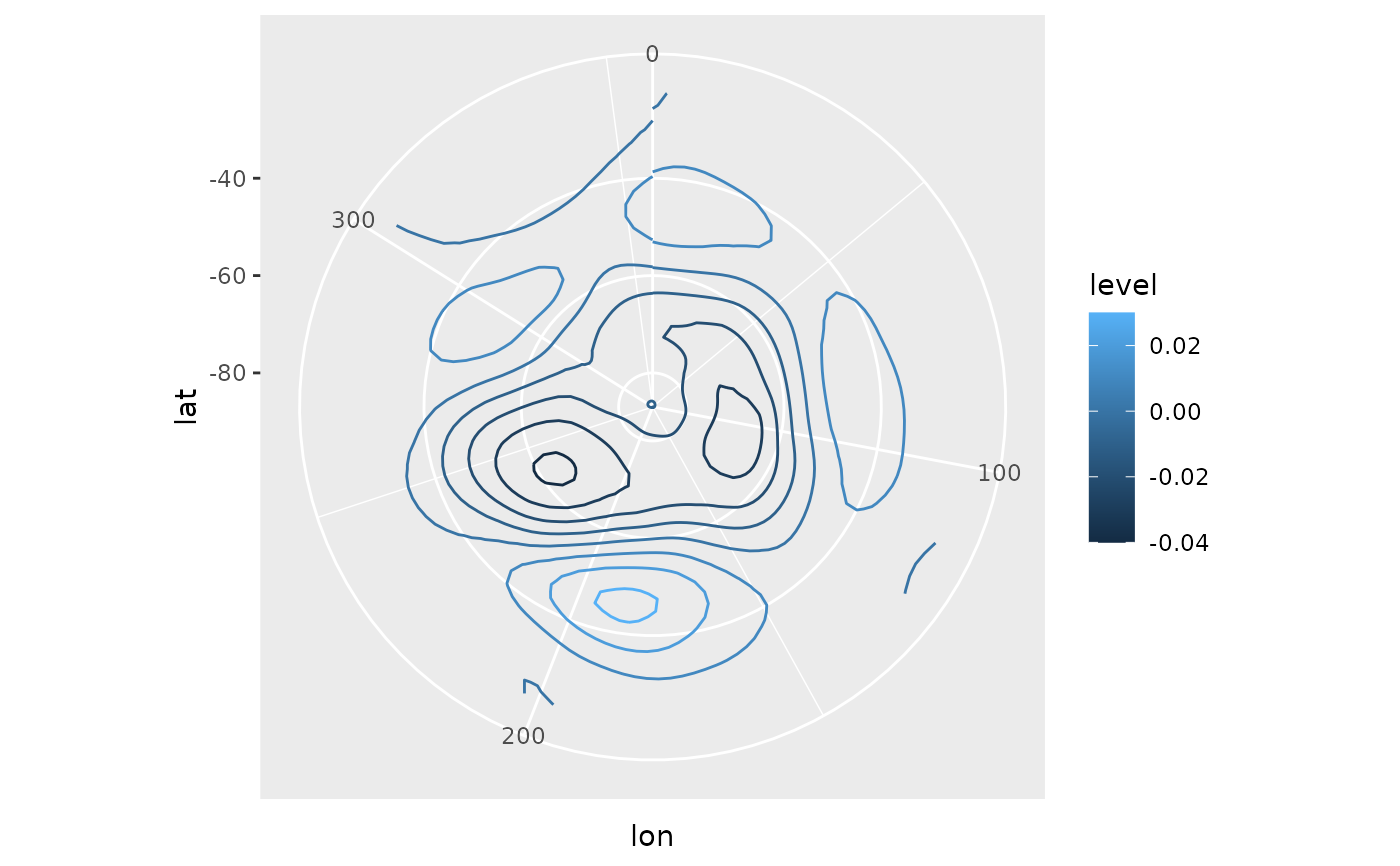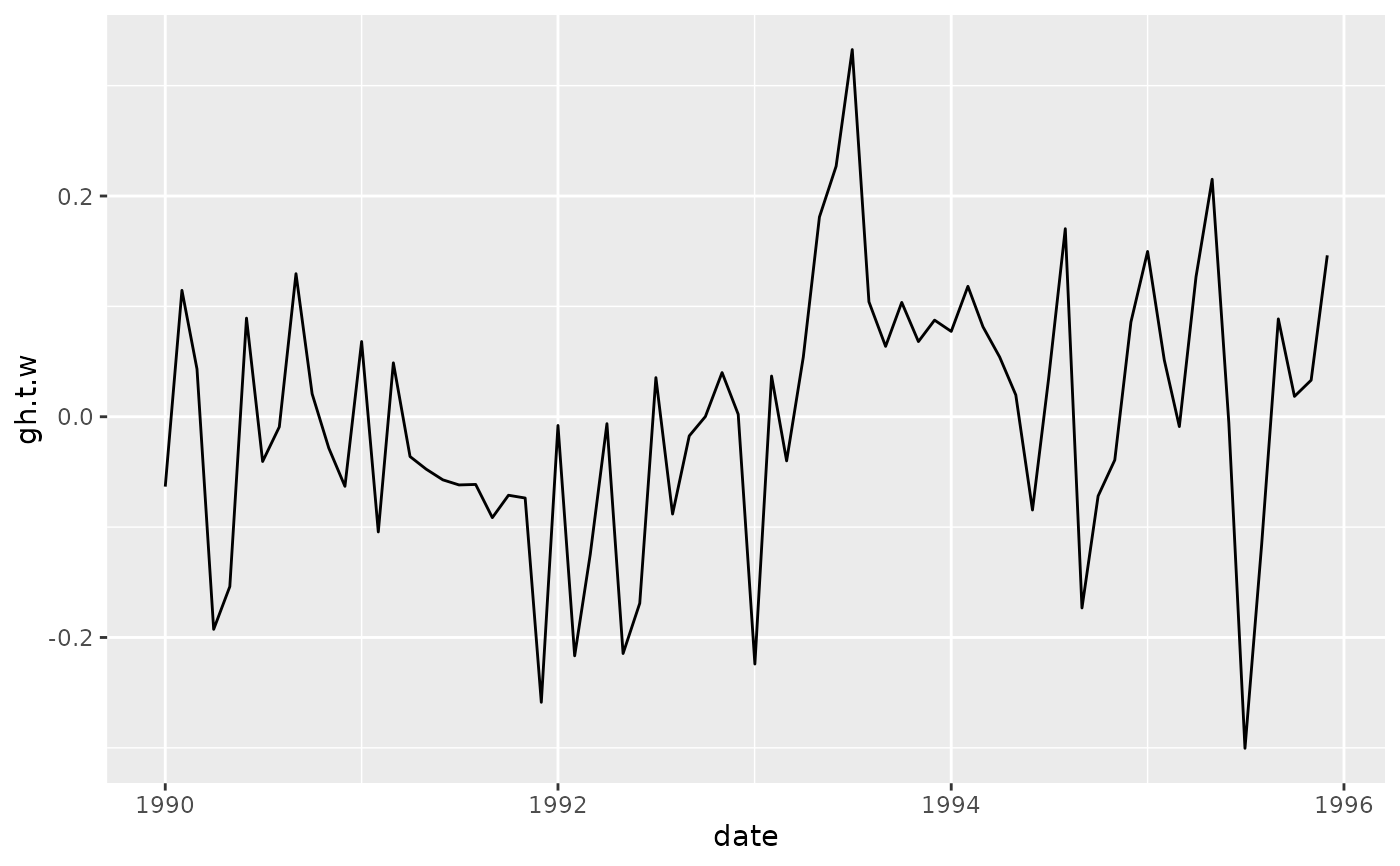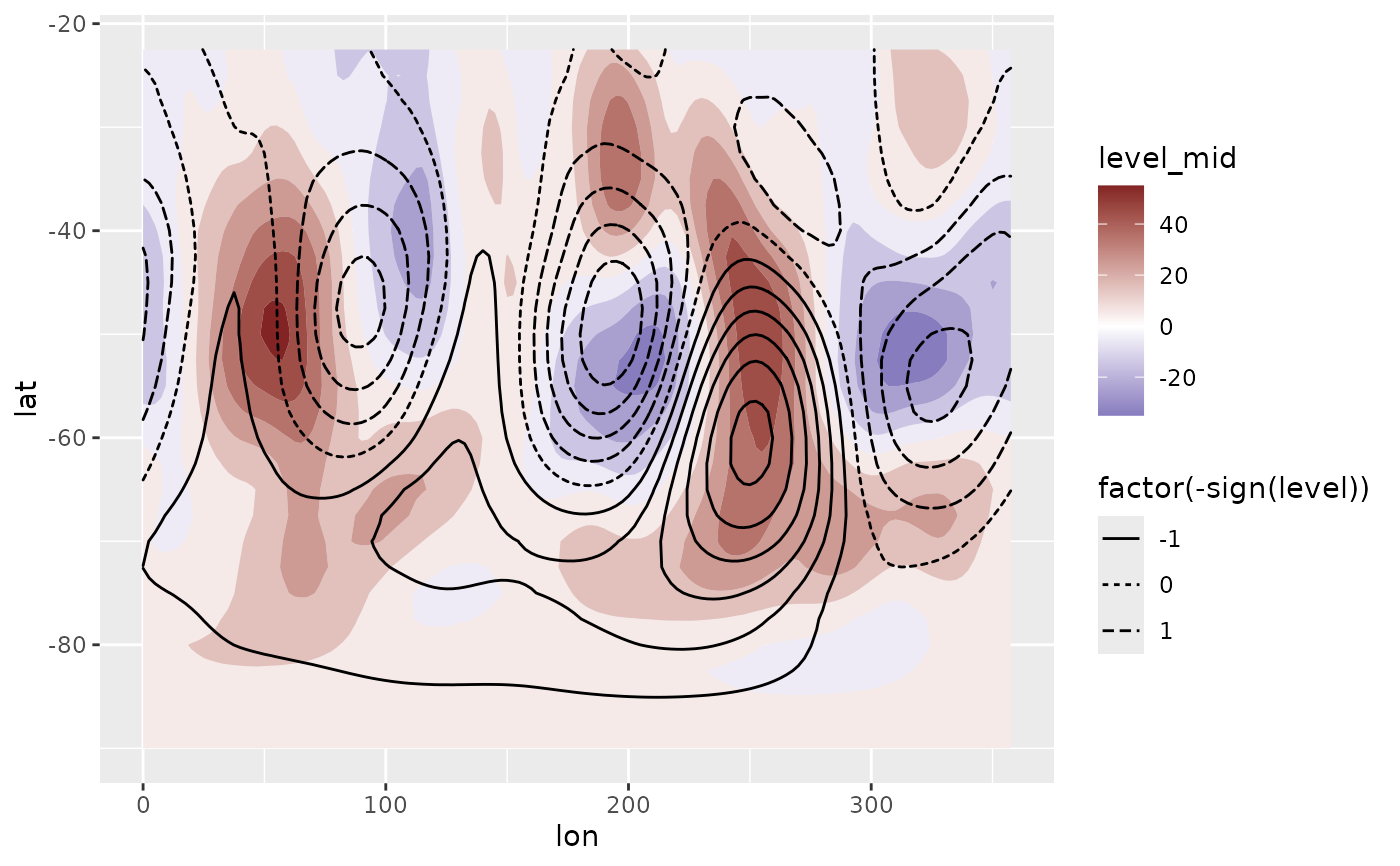Computes Singular Value Decomposition (also known as Principal Components Analysis or Empirical Orthogonal Functions).

EOF(
formula,
n = 1,
data = NULL,
B = 0,
probs = c(lower = 0.025, mid = 0.5, upper = 0.975),
rotate = FALSE,
suffix = "PC",
fill = NULL,
engine = NULL
)

## Arguments

formula

a formula to build the matrix that will be used in the SVD decomposition (see Details)

n

which singular values to return (if NULL, returns all)

data

a data.frame

B

number of bootstrap samples used to estimate confidence intervals. Ignored if <= 1.

probs

the probabilities of the lower and upper values of estimated confidence intervals. If named, it's names will be used as column names.

rotate

if TRUE, scores and loadings will be rotated using varimax

suffix

character to name the principal components

fill

value to infill implicit missing values or NULL if the data is dense.

engine

function to use to compute SVD. If NULL it uses irlba::irlba (if installed) if the largest singular value to compute is lower than half the maximum possible value, otherwise it uses base::svd. If the user provides a function, it needs to be a drop-in replacement for base::svd (the same arguments and output format).

## Value

An eof object which is just a named list of data.tables

left

data.table with left singular vectors

right

data.table with right singular vectors

sdev

data.table with singular values, their explained variance, and, optionally, quantiles estimated via bootstrap

There are some methods implemented

## Details

Singular values can be computed over matrices so formula denotes how to build a matrix from the data. It is a formula of the form VAR ~ LEFT | RIGHT (see Formula::Formula) in which VAR is the variable whose values will populate the matrix, and LEFT represent the variables used to make the rows and RIGHT, the columns of the matrix. Think it like "VAR as a function of LEFT and RIGHT". The variable combination used in this formula must identify an unique value in a cell.

So, for example, v ~ x + y | t would mean that there is one value of v for each combination of x, y and t, and that there will be one row for each combination of x and y and one row for each t.

In the result, the left and right vectors have dimensions of the LEFT and RIGHT part of the formula, respectively.

It is much faster to compute only some singular vectors, so is advisable not to set n to NULL. If the irlba package is installed, EOF uses irlba::irlba instead of base::svd since it's much faster.

The bootstrapping procedure follows Fisher et.al. (2016) and returns the standard deviation of each singular value.

Other meteorology functions: Derivate(), GeostrophicWind(), WaveFlux(), thermodynamics, waves

## Examples

data.table::setDTthreads(1)
# The Antarctic Oscillation is computed from the
# monthly geopotential height anomalies weighted by latitude.
library(data.table)
data(geopotential)
geopotential <- copy(geopotential)
geopotential[, gh.t.w := Anomaly(gh)*sqrt(cos(lat*pi/180)),
by = .(lon, lat, month(date))]
#>           lon   lat lev       gh       date        gh.t.w
#>      1:   0.0 -22.5 700 3163.839 1990-01-01 -3.824174e+00
#>      2:   2.5 -22.5 700 3162.516 1990-01-01 -3.591582e+00
#>      3:   5.0 -22.5 700 3162.226 1990-01-01 -2.862909e+00
#>      4:   7.5 -22.5 700 3162.323 1990-01-01 -2.403045e+00
#>      5:  10.0 -22.5 700 3163.097 1990-01-01 -2.067122e+00
#>     ---
#> 290300: 347.5 -90.0 700 2671.484 1995-12-01 -1.449750e-07
#> 290301: 350.0 -90.0 700 2671.484 1995-12-01 -1.449750e-07
#> 290302: 352.5 -90.0 700 2671.484 1995-12-01 -1.449750e-07
#> 290303: 355.0 -90.0 700 2671.484 1995-12-01 -1.449750e-07
#> 290304: 357.5 -90.0 700 2671.484 1995-12-01 -1.449750e-07

eof <- EOF(gh.t.w ~ lat + lon | date, 1:5, data = geopotential,
B = 100, probs = c(low = 0.1, hig = 0.9))

# Inspect the explained variance of each component
summary(eof)
#> Importance of components:
#> Component Explained variance Cumulative variance
#>       1                   32%                 32%
#>       2                   11%                 43%
#>       3                    8%                 51%
#>       4                    7%                 58%
#>       5                    6%                 64%
screeplot(eof)# Keep only the 1st.
aao <- cut(eof, 1)

# AAO field
library(ggplot2)
ggplot(aao$left, aes(lon, lat, z = gh.t.w)) + geom_contour(aes(color = after_stat(level))) + coord_polar()# AAO signal ggplot(aao$right, aes(date, gh.t.w)) +
geom_line()# standard deviation, % of explained variance and
# confidence intervals.
aao\$sdev
#>     PC       sd        r2     low      hig
#> 1: PC1 7050.352 0.3176266 6284.91 7899.572

# Reconstructed fields based only on the two first
# principal components
field <- predict(eof, 1:2)

# Compare it to the real field.
ggplot(field[date == date], aes(lon, lat)) +
geom_contour_fill(aes(z = gh.t.w), data = geopotential[date == date]) +
geom_contour2(aes(z = gh.t.w, linetype = factor(-sign(stat(level))))) +
scale_fill_divergent()
#> Warning: stat(level) was deprecated in ggplot2 3.4.0.
#> ℹ Please use after_stat(level) instead.